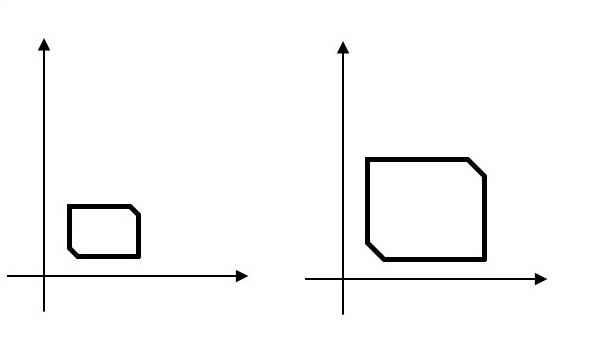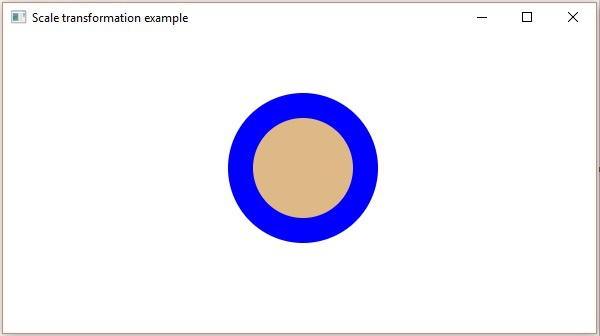# JavaFX - Scaling Transformation

To change the size of an object, scaling transformation is used. In the scaling process, you either expand or compress the dimensions of the object. Scaling can be achieved by multiplying the original coordinates of the object with the scaling factor to get the desired result.## Example

Following is the program which demonstrates scaling in JavaFX. Here, we are creating 2 circles (nodes) at the same location with the same dimensions, but with different colors (Blurywood and Blue). We are also applying scaling transformation on the circle with a blue color.

Save this code in a file with the name ScalingExample.java.

```import javafx.application.Application;
import javafx.scene.Group;
import javafx.scene.Scene;
import javafx.scene.paint.Color;
import javafx.scene.shape.Circle;
import javafx.scene.transform.Scale;
import javafx.stage.Stage;

public class ScalingExample extends Application {
@Override
public void start(Stage stage) {
//Drawing Circle1
Circle circle1 = new Circle(300, 135, 50);

//Setting the color of the circle
circle1.setFill(Color.BLUE);

//Setting the stroke width of the circle
circle1.setStrokeWidth(20);

//Drawing Circle2
Circle circle2 = new Circle(300, 135, 50);

//Setting the color of the circle
circle2.setFill(Color.BURLYWOOD);

//Setting the stroke width of the circle
circle2.setStrokeWidth(20);

//Creating the scale transformation
Scale scale = new Scale();

//Setting the dimensions for the transformation
scale.setX(1.5);
scale.setY(1.5);

//Setting the pivot point for the transformation
scale.setPivotX(300);
scale.setPivotY(135);

//Adding the scale transformation to circle1

//Creating a Group object
Group root = new Group(circle1, circle2);

//Creating a scene object
Scene scene = new Scene(root, 600, 300);

//Setting title to the Stage
stage.setTitle("Scaling transformation example");

stage.setScene(scene);

//Displaying the contents of the stage
stage.show();
}
public static void main(String args[]){
launch(args);
}
}
```

Compile and execute the saved java file from the command prompt using the following commands.

```javac ScalingExample.java
java ScalingExample
```

On executing, the above program generates a JavaFX window as shown below.javafx_transformations.htm